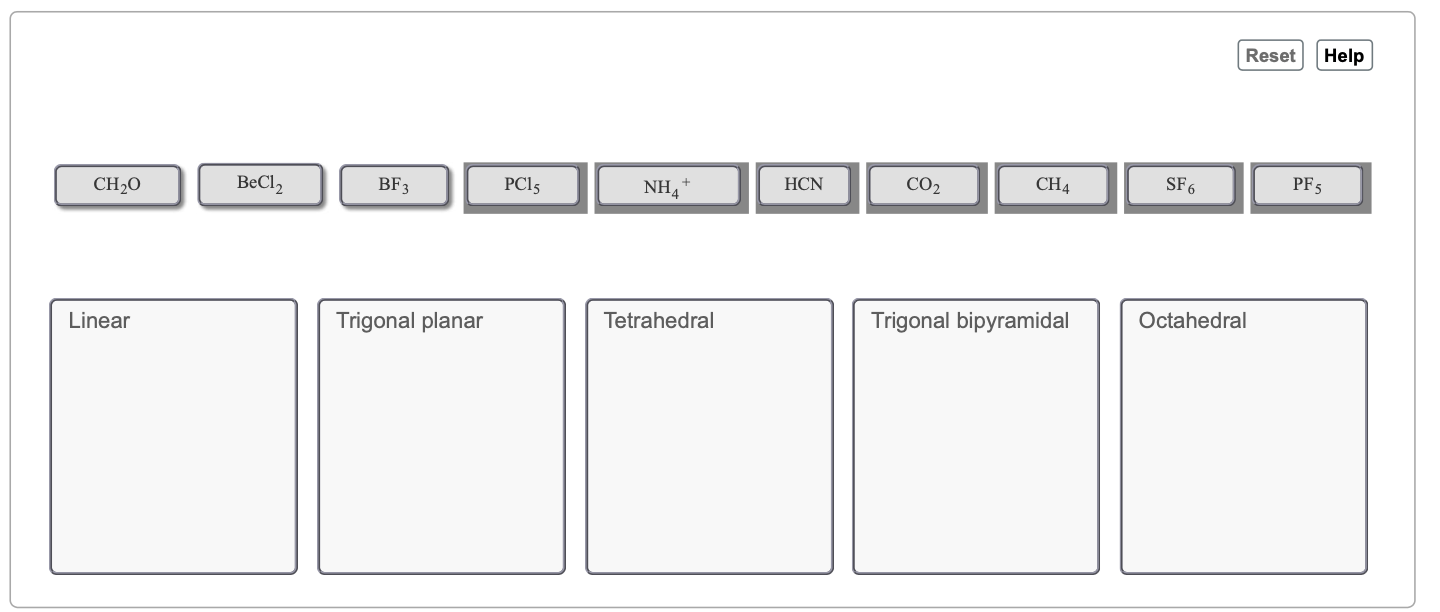# Problem: Part B. Group the following molecular formulas under their respective geometries. Drag the appropriate items to their respective bins.Since notations and molecular formulas are directly relatable, the geometries of molecules can be predicted directly from the molecular formula. Recall that AB4 can represent CH4, which is methane, and its geometry can be predicted as tetrahedral. As it turns out, methane and other AB4-type molecules (that do not have additional electrons via nonbonding pairs) exhibit tetrahedral geometry. None of the molecules in the simulation contain nonbonding pairs of electrons.

###### FREE Expert Solution
89% (193 ratings)View Complete Written Solution
###### Problem Details

Part B. Group the following molecular formulas under their respective geometries.Drag the appropriate items to their respective bins.

Since notations and molecular formulas are directly relatable, the geometries of molecules can be predicted directly from the molecular formula. Recall that AB4 can represent CH4, which is methane, and its geometry can be predicted as tetrahedral. As it turns out, methane and other AB4-type molecules (that do not have additional electrons via nonbonding pairs) exhibit tetrahedral geometry. None of the molecules in the simulation contain nonbonding pairs of electrons.